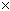SEARCH HOMEMath Central Quandaries & QueriesQuestion from Jimi, a student: The new businessperson hopes to earn $50,000 per year. The profit percentage for this type of business is typically 10%. How much must sales be?Hi Jimi. Ten percent of the sales is the profit, which becomes her earnings. So we can solve this with algebra. Let S = the amount of sales she must have. 10%S =$50 000.

We want to find the value of S, so we need to make the left side of the equation just S (this is called isolating S). That means we have to get the 10% out of the left side of the equation. To do that, we will divide by 10% on both sides of the equation. Then the 10% cancels out on the left side:

10%S / 10% = $50 000 / 10% therefore S =$50 000 / 10%

Now remember that percent means per hundred, so 10% means 10 / 100, which is 0.10. So we can write it like this:

S = \$50 000 / 0.10

Can you finish the question now?
Stephen La Rocque.Math Central is supported by the University of Regina and The Pacific Institute for the Mathematical Sciences.